# 20194220 2019-2020-2 《Python程序设计》实验二报告

## 2. 实验过程及结果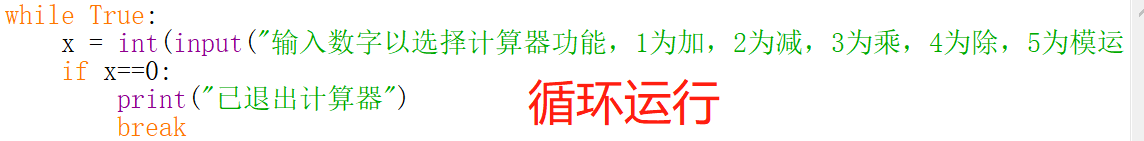↓ 2进制 8进制 10进制 16进制
2进制 - bin(int(n,8)) bin(int(n,10)) bin(int(n,16))
8进制 oct(int(n,2)) - oct(int(n,10)) oct(int(n,16))
10进制 int(n,2) int(n,8) - int(n,16)
16进制 hex(int(n,2)) hex(int(n,8)) hex(int(n,10)) -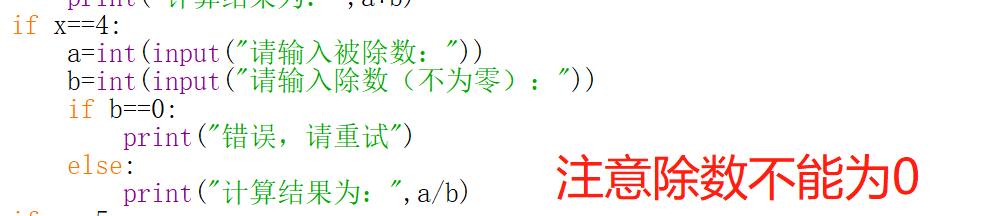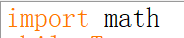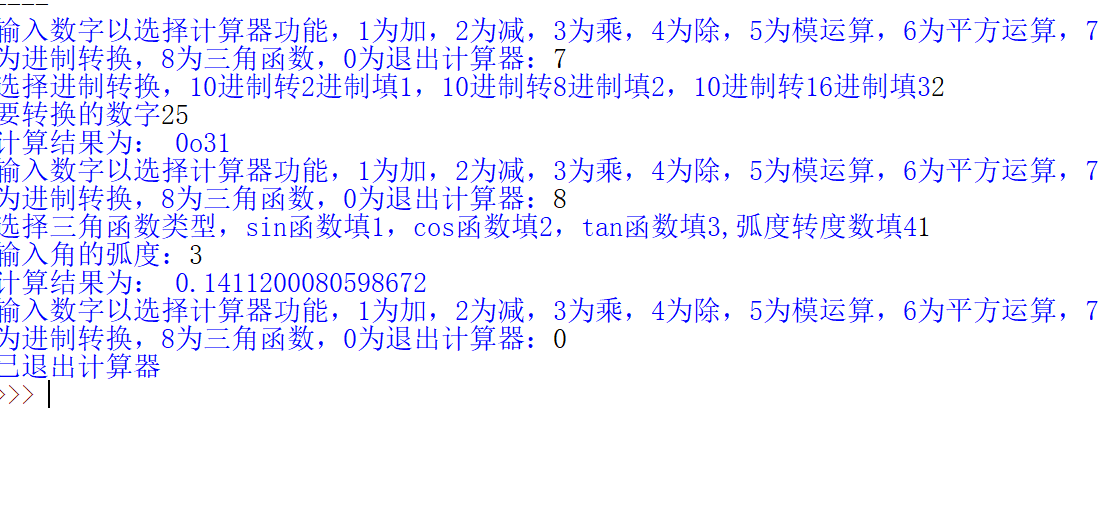import math
while True:
x = int(input("输入数字以选择计算器功能，1为加，2为减，3为乘，4为除，5为模运算，6为平方运算，7为进制转换，8为三角函数，0为退出计算器："))
if x==0:
print("已退出计算器")
break
if x==1:
a=int(input("请输入第一个加数："))
b=int(input("请输入第二个加数："))
print("计算结果为：",a+b)
if x==2:
a=int(input("请输入被减数："))
b=int(input("请输入减数："))
print("计算结果为：",a-b)
if x==3:
a=int(input("请输入第一个乘数："))
b=int(input("请输入第二个乘数："))
print("计算结果为：",a*b)
if x==4:
a=int(input("请输入被除数："))
b=int(input("请输入除数（不为零）："))
if b==0:
print("错误，请重试")
else:
print("计算结果为：",a/b)
if x==5:
a=int(input("请输入第一个数字："))
b=int(input("请输入第二个数字："))
print("计算结果为：",a%b)
if x==6:
a=int(input("请输入底数："))
b=int(input("请输入幂："))
print("计算结果为：",a**b)
if x==7:
y = int(input("选择进制转换，10进制转2进制填1，10进制转8进制填2，10进制转16进制填3"))
if y==1:
a = int(input("要转换的数字"))
b = (bin(a))
print("计算结果为：",b)
if y==2:
a = int(input("要转换的数字"))
b = (oct(a))
print("计算结果为：",b)
if y==3:
a = int(input("要转换的数字"))
b = (hex(a))
print("计算结果为：",b)
if x==8:
y = int(input("选择三角函数类型，sin函数填1，cos函数填2，tan函数填3,弧度转度数填4"))
if y==1:
a = int(input("输入角的弧度："))
b = math.sin(a)
print("计算结果为：",b)
if y==2:
a = int(input("输入角的弧度："))
b = math.sin(a)
print("计算结果为：",b)
if y==3:
a = int(input("输入角的弧度："))
b = math.tan(a)
print("计算结果为：",b)
if y==4:
a = int(input("输入角的弧度："))
b = math.degrees(a)
print("计算结果为：",b)



## 3. 实验过程中遇到的问题和解决过程

• 问题1：忘记了= 和==的区别
• 问题1解决方案：牢记=是赋值，==才是等于
• 问题2：def和直接逻辑运算的选择
• 问题2解决方案：没有重复的运用加法或者减法，于是就不使用def(),而是直接使用了逻辑运算
-问题3：在用到数字的时候忘记字符串和int数的区别，导致一直运行不起来
-问题3解决方案：百度搜索，查找资料（以下实例）在y= 的后面要加上int()转换，不然下面输入的数字类型对不上号，运行不起来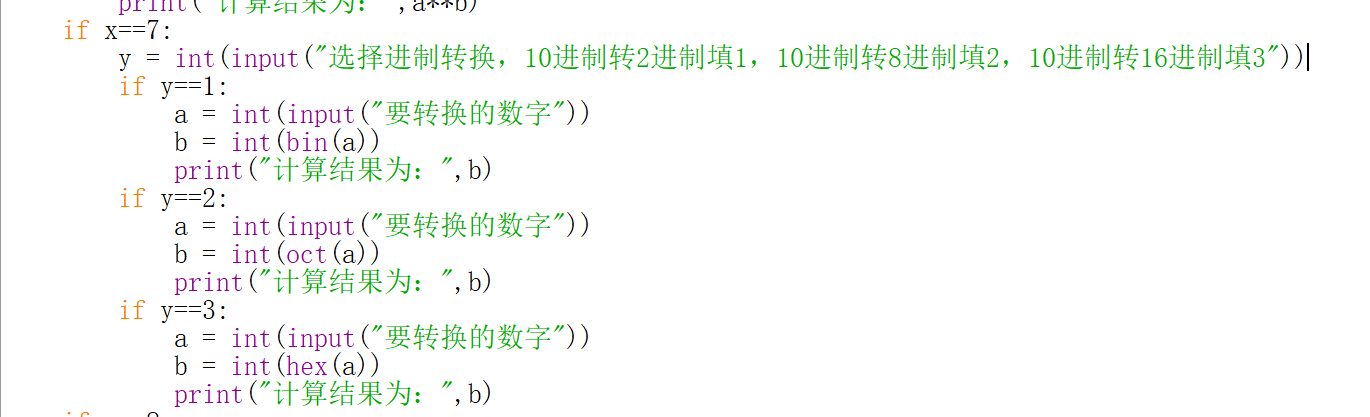## 其他（感悟、思考等）

posted @ 2020-04-11 17:44  20194220梁勇  阅读(133)  评论(0编辑  收藏